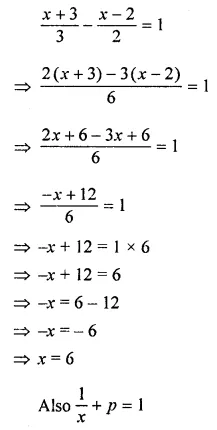## ML Aggarwal Class 8 Solutions for ICSE Maths Chapter 12 Linear Equations and Inequalities in one Variable Ex 12.1

Solve the following equations (1 to 12):
Question 1.
(i) 5x – 3 = 3x – 5
(ii) 3x – 7 = 3(5 – x)
Solution: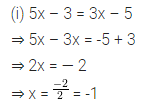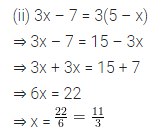Question 2.
(i) 4(2x + 1) = 3(x – 1) + 7
(ii) 3(2p – 1) = 5 – (3p – 2)
Solution: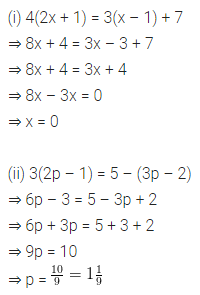Question 3.
(i) 5y – 2[y – 3(y – 5)] = 6
(ii) 0.3(6 – x) = 0.4(x + 8)
Solution:Question 4.
(i) $$\frac{x-1}{3}=\frac{x+2}{6}+3$$
(ii) $$\frac{x+7}{3}=1+\frac{3 x-2}{5}$$
Solution: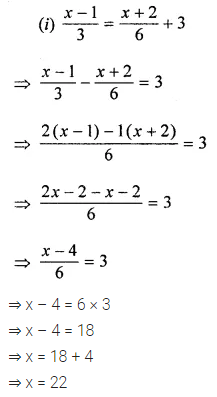Question 5.Solution: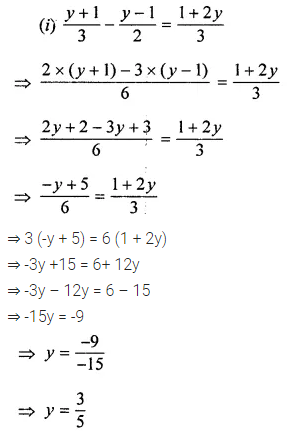Question 6.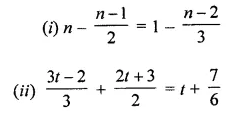Solution: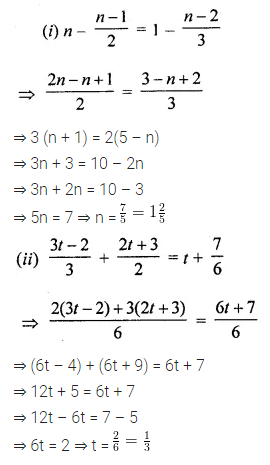Question 7.
(i) 4(3x + 2) – 5(6x – 1) = 2(x – 8) – 6(7x – 4)
(ii) 3(5x + 7) + 5(2x – 11) = 3(8x – 5) – 15
Solution:Question 8.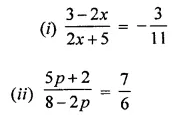Solution:Question 9.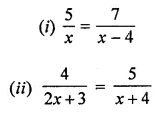Solution: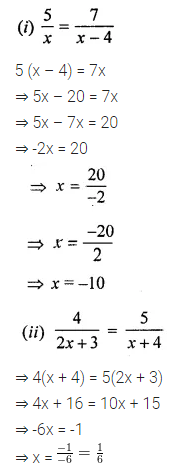Question 10.Solution:Question 11.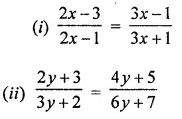Solution: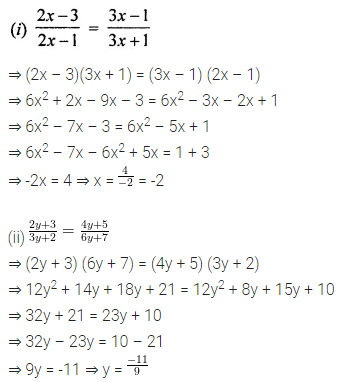Question 12.
If x = p + 1, find the value of p from the equation $$\frac{1}{2}$$ (5x – 30) – $$\frac{1}{3}$$ (1 + 7p) = $$\frac{1}{4}$$
Solution:Question 13.
Solve $$\frac{x+3}{3}-\frac{x-2}{2}=1$$, Hence find p if $$\frac{1}{x}+p$$ = 1.
Solution: